JMSLTM Numerical Library 7.2.0
com.imsl.math

Class CsSmooth

• All Implemented Interfaces:
Serializable, Cloneable

public class CsSmooth
extends Spline
Extension of the Spline class to construct a smooth cubic spline from noisy data points.

Class CsSmooth is designed to produce a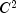cubic spline approximation to a data set in which the function values are noisy. This spline is called a smoothing spline. It is a natural cubic spline with knots at all the data abscissas x = xData, but it does not interpolate the data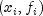. The smoothing spline S is the uniquefunction that minimizes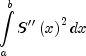subject to the constraint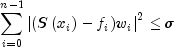whereis the smoothing parameter. The reader should consult Reinsch (1967) for more information concerning smoothing splines. CsSmooth solves the above problem when the user provides the smoothing parameter. CsSmoothC2 attempts to find the "optimal" smoothing parameter using the statistical technique known as cross-validation. This means that (in a very rough sense) one chooses the value ofso that the smoothing spline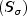best approximates the value of the data at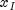, if it is computed using all the data except the i-th; this is true for all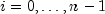. For more information on this topic, we refer the reader to Craven and Wahba (1979).

Example, Serialized Form
• Constructor Detail

• CsSmooth

public CsSmooth(double[] xData,
double[] yData)
Constructs a smooth cubic spline from noisy data using cross-validation to estimate the smoothing parameter. All of the points have equal weights.
Parameters:
xData - A double array containing the x-coordinates of the data. Values must be distinct.
yData - A double array containing the y-coordinates of the data. The arrays xData and yData must have the same length.
• CsSmooth

public CsSmooth(double[] xData,
double[] yData,
double[] weight)
Constructs a smooth cubic spline from noisy data using cross-validation to estimate the smoothing parameter. Weights are supplied by the user.
Parameters:
xData - A double array containing the x-coordinates of the data. Values must be distinct.
yData - A double array containing the y-coordinates of the data. The arrays xData and yData must have the same length.
weight - A double array containing the relative weights. This array must have the same length as xData.
JMSLTM Numerical Library 7.2.0